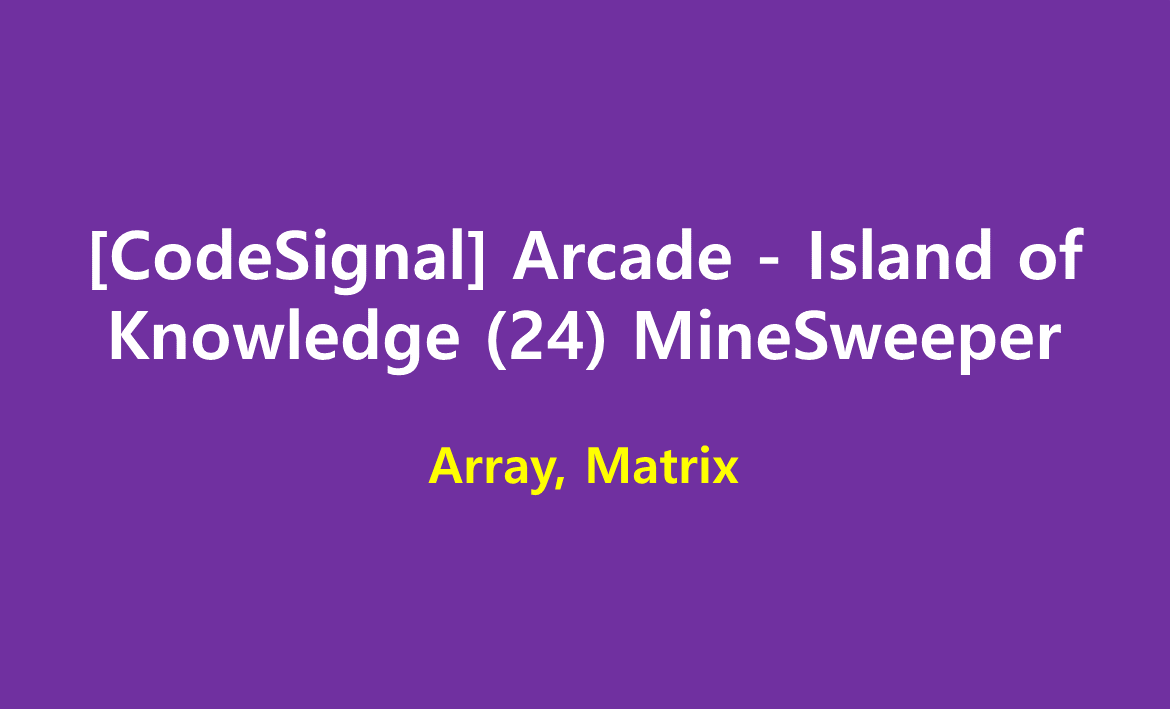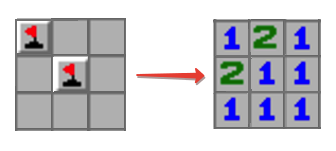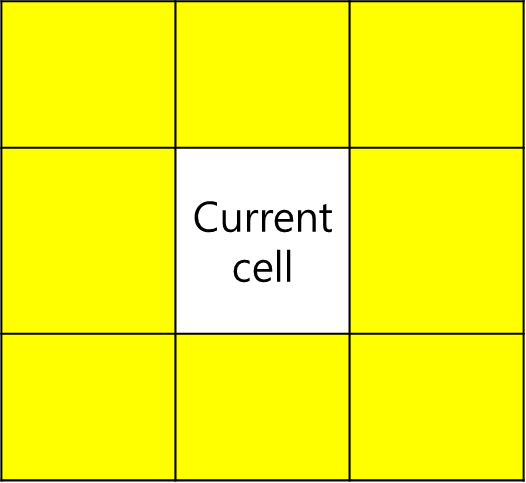# [CodeSignal] Arcade - Island of Knowledge (25) MineSweeper

limelimejiwon·2022년 5월 24일
0

## Python으로 공부하는 코딩테스트

목록 보기
66/67## 📄 Description

In the popular Minesweeper game you have a board with some mines and those cells that don't contain a mine have a number in it that indicates the total number of mines in the neighboring cells. Starting off with some arrangement of mines we want to create a Minesweeper game setup.

### Example

For

matrix = [[true, false, false],
[false, true, false],
[false, false, false]]

the output should be

solution(matrix) = [[1, 2, 1],
[2, 1, 1],
[1, 1, 1]]

Check out the image below for better understanding:### 📌 Input/Output

• [execution time limit] 4 seconds (py3)

• [input] array.array.boolean matrix

A non-empty rectangular matrix consisting of boolean values - true if the corresponding cell contains a mine, false otherwise.

### 📌 Guaranteed constraints:

2 ≤ matrix.length ≤ 100,
2 ≤ matrix.length ≤ 100.

• [output] array.array.integer

Rectangular matrix of the same size as matrix each cell of which contains an integer equal to the number of mines in the neighboring cells. Two cells are called neighboring if they share at least one corner.

## 💭 How to solve

### Below is the neighbor cells• count mine(which is True in the array) in the neighbor cells.
• you have to check if the cell is valid one.

## 💻 My Submission

def solution(matrix):
r, c=len(matrix),len(matrix)
for i in range(r):
ans=[]
for j in range(c):
mine=0
return answer